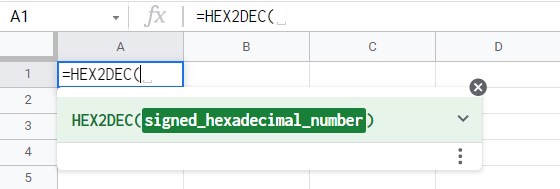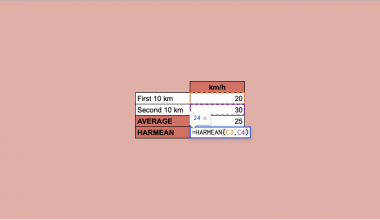# How to Use HEX2DEC Function in Google Sheets

The HEX2DEC function in Google Sheets is useful when you need to convert a hexadecimal value to a decimal number.

The hexadecimal numbering system is extensively utilized in the world of engineering and computing. It is often used to describe locations in computer memory. Also, hexadecimal digits are sometimes used as color codes on the web. In many cases, though, non-programmers find decimal numbers easier and more convenient.

Although there is a formula to convert a hexadecimal number to decimal, it is quite tricky and time-consuming. Unless you’re a math maniac, surely you wouldn’t want to manually convert hundreds or even thousands of hexadecimal numbers to decimal. Here’s where the `HEX2DEC` function in Google Sheets can come in handy.

The `HEX2DEC` function takes a hexadecimal number as an argument and automatically transforms it to its decimal counterpart. Thanks to this function, you won’t be scratching your head anymore in computing the value you need.

## The Anatomy of the HEX2DEC Function

For you to use the `HEX2DEC` function, you must understand its syntax first. Here’s how you should write it:

As you can see, the HEX2DEC function is quite simple. Here are the components:

• = we need to type in the equal sign first every time we use a function or formula.
• HEX2DEC() this is our `HEX2DEC` function.
• signed_hexadecimal_number is the only required parameter of `HEX2DEC` function. This parameter should hold the value you need to convert into decimal.

## A Real Example of Using HEX2DEC Function

Earlier, you’ve seen how easy the `HEX2DEC` function is. At this point, let’s utilize this function in a real-world setting.

Let’s consider this example.

Kevin is developing a program that requires decimal values to be imported from a spreadsheet. He was already given a spreadsheet to start with, but it turned out that the spreadsheet only contains hexadecimal values. For the program to work properly, he must convert the values into decimal.Although he can write a code on the program to do the conversion, it would be more efficient to convert them on the spreadsheet directly. To do this, he added a column on the spreadsheet and utilized the `HEX2DEC` function, transforming the hexadecimal values into their decimal format.Now, Kevin doesn’t need to write a complicated formula on the program just so he can convert and import decimal values.

If you want to have a copy of Kevin’s spreadsheet so that you can play with the `HEX2DEC` function, just click the link below.

In the next section, you will learn how to write this function in Google Sheets.

## How to Use HEX2DEC Function in Google Sheets

1. First, open the spreadsheet with the hexadecimal values that you need to convert. Alternatively, you can make a copy of our example spreadsheet by clicking the link above.2. With the spreadsheet already open, double-click the cell where you need to input the HEX2DEC function. Cell B2 is selected in the example below.3. Next, initiate the function by typing in the equal sign ‘=’, followed by ‘HEX2DEC(’.4. At this point, you need to specify its only parameter, which is signed_hexadecimal_number. Type in the hexadecimal value you want to convert into decimal. You can also use the cell reference of the value if it’s already on the spreadsheet, like in the case below.5. Now that you have specified the parameter, complete the function by typing in the close parenthesis ‘)’. Then, hit the Enter key on your keyboard.6. To recreate the `HEX2DEC` function in the succeeding cells, you can use the autofill feature. Select B2, then drag the small blue box on the bottom right corner over the adjacent cells.Great work! Now you know how to write a basic `HEX2DEC` function in Google Sheets.

## Using the HEX2DEC Function with Another Function

`HEX2DEC` can also be used along with other functions in Google Sheets. In this section, you’ll learn how to combine it with the `CHAR` function to generate symbols or characters in your spreadsheet.

1. Start by opening a new Google Sheets spreadsheet or use the one from our activity earlier.
2. Next, double-click on any cell where you want to display the output. In the example below, cell A1 is selected.3. This time, type in ‘=HEX2DEC(’ to initiate the function.4. For the parameter, we’ll enter the hexadecimal value ‘A9’. Take note that we’ll be entering an actual value and not the cell reference A9. To do this, type in ‘“A9”’ (with double quotes) as the parameter.5. Then, type in the close parenthesis ‘)’ and hit Enter to display the output. You should now see the decimal value, which is 169, in your spreadsheet.6. Now, let’s transform this decimal number into its character counterpart using the `CHAR` function. Access the previous function and include ‘CHAR(’ right after the equal sign. Afterward, make the whole `HEX2DEC` function as its parameter. Be guided by the image below.7. Finally, hit Enter on your keyboard to show the result.That’s pretty cool! We just used HEX2DEC together with the CHAR function to generate a copyright symbol ©.

## Points to Consider When Using HEX2DEC Function in Google Sheets

• Since the `HEX2DEC` function only uses hexadecimal values, only letters A to F and digits (0-9) are valid. Using characters other than those specified will cause the function to return an error.
• Hexadecimal values are not case-sensitive. This means that A-F and a-f are equivalent.
• The `HEX2DEC` function can have a maximum hexadecimal value of 7fffffffff and a minimum of 8000000000.
• If the parameter provided is a valid hexadecimal value, it will be converted to the appropriate string input automatically. For instance, HEX2DEC(120) is equivalent to HEX2DEC(“120”).

That’s essentially it for `HEX2DEC` in Google Sheets! It’s a fairly straightforward function with a very simple application. You just learned how to write its basic syntax and how you can use it with another function. Make sure to check out our other articles in Google Sheets to learn other powerful functions.Our goal this year is to create lots of rich, bite-sized tutorials for Google Sheets users like you. If you liked this one, you'll love what we are working on! Readers receive ✨ early access ✨ to new content.

##### You May Also Like## How to Create Candlestick Chart in Google Sheets

A candlestick chart is a visualization tool available in Google Sheets that helps track movements in the value…## How to Use HARMEAN Function in Google Sheets

The HARMEAN function in Google Sheets is used to calculate the harmonic mean of a dataset.  The harmonic…## How to Use GESTEP Function in Google Sheets

The GESTEP function in Google Sheets is useful for Engineering purposes, as it determines a rate is strictly…## How to Use the DMIN Function in Google Sheets

The DMIN Function in Google Sheets is useful when you want to get the minimum value in a…